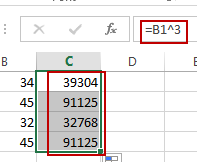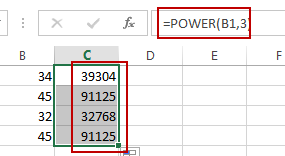# How to Calculate Exponential Value for a Range of Cells in Excel

This post will guide you how to calculate the exponential values for a range of cells in Excel. How to use the POWER function to do exponential calculation to a range of cells in Excel. How do I use the symbol ^ to calculate the exponential value for a range of cells in Excel. How to generate exponential series of values with known base number and exponent number in Excel.

Assuming that you have a list of data in range B1:B4 which contain numbers, and you want to calculate exponential value for those numbers in Excel. How to achieve it. You can use the symbol ^ or Power function to achieve the result.

## Calculate Exponential Value for a Range of Cells with Symbol ^

If you want the number 10 multiplied by a power of 3 in excel, it is the same as 10x10x10. You can use the symbol ^ operator to achieve the result. Just run the formula=10^3 in a blank cell. Let’s do the following steps to calculate the exponential value for the numbers in Range B1:B4 to multiply by a power of 3.

#1 select one blank cell in column C, and type the following formula in Cell C1.

=B1^3

#2 press Enter key in your keyboard, and then drag the AutoFill Handle over other cells to apply this formula.## Calculate Exponential Value for a Range of Cells with Power Function

You can also use the POWER function to achieve the same result of calculate exponential value for a range of cells in Excel. Just type the following formula in blank cell C1, and press Enter key, and then drag the AutoFill Handle down to other cells to apply this formula.

=POWER(B1,3)Video: Calculate Exponential Value for a Range of Cells

### Related Functions

• Excel POWER function
The Excel POWER function returns the result of a number raised to a given power.The syntax of the POWER function is as below:= POWER (number,power)….